04.10.2023

# Comparison of a Creep-Only Wellbore Model with an Analytical Creep SolutionIn this study, we compare the creep model used in Elfen to an analytical solution developed by Barker.

### Barker formulation

The analytical solution predicts the closure rate of an open hole well assuming:

• The stress distribution around the wellbore remains elastic,
• The deviatoric stress is constant

According to the Barker formulation, the radius of the well as a function of time is expressed as: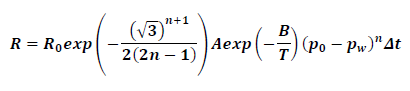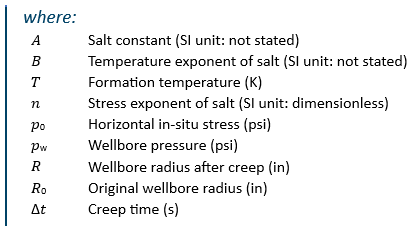### Elfen creep model

The Munson-Dawson steady-state model for the creep of salt is implemented in Elfen and captures both short- and long-term effects. The steady-state model creep rate according to the Munson-Dawson model is given as: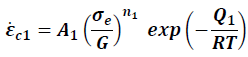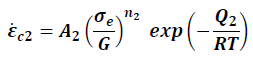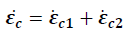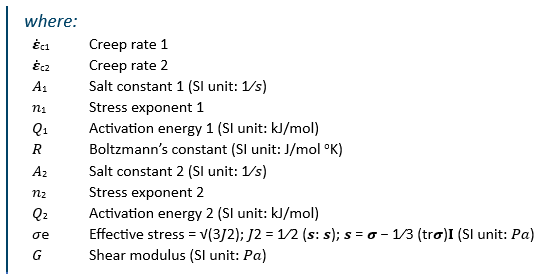The Elfen analytical creep formulation can be reduced to Barker’s solution by:

• Accounting for a single creep component (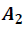= 0 )
• Assuming constant effective stress within the salt
• Appropriate setting of coefficients

### Model set-up for Numerical Assessment

This model features

• 3D - pseudo plane strain
• Uniform compaction
• The salt is pre-stressed to in situ values, with balancing in situ stresses applied to the far-field boundary
• For direct comparison to the analytical model, contact between salt and casing is not considered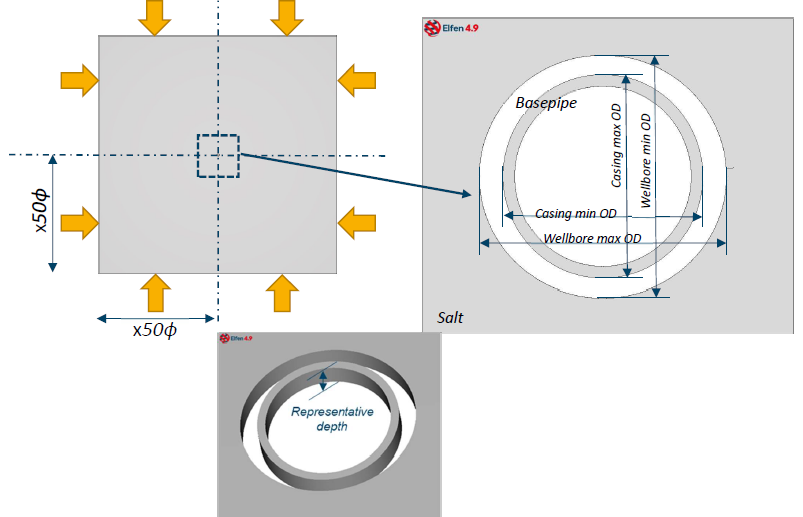Figure 1: Salt Creep Model

### Results and comparison

The results below compare the analytical and numerical solutions of wellbore closure due to creep assuming the conditions as listed in Table 2-1. The comparison are broken down into:

1. Comparison of the two analytical solutions (Barker and Elfen),
2. Comparison of the analytical and numerical solutions.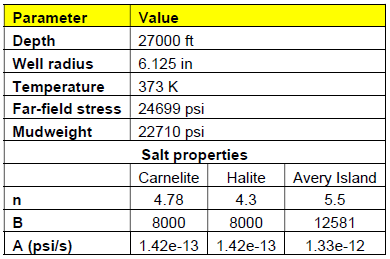Model parameters and salt properties for creeping wellbore closure assessment

### Numerical vs. Analytical (Barker vs. Elfen)

Figure 2 displays the analytical solution for wellbore closure comparing the Barker and Elfen solution for three types of salt. Evidently the solutions are in agreement.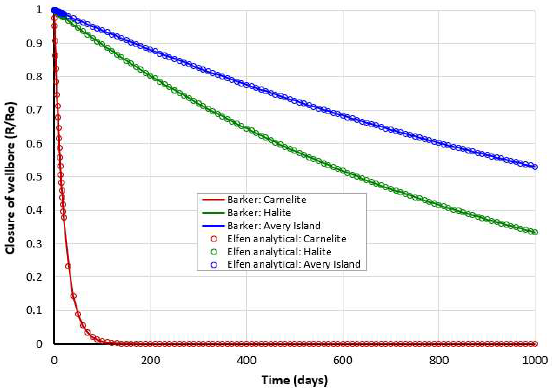Figure 2: Barker’s solution for Carnelite, Halite and Avery Island compared against Elfen analytical solution

The analytical solution assumes constant effective stress, however as the wellbore creeps the local effective stress around the wellbore would diminish.

To evaluate the effect of varying effective stress within the salt, a set of numerical simulations were performed; as for the Elfen analytical solution, the Elfen numerical solution only considers a single component of creep. Figure 3 compares the numerical and analytical solution.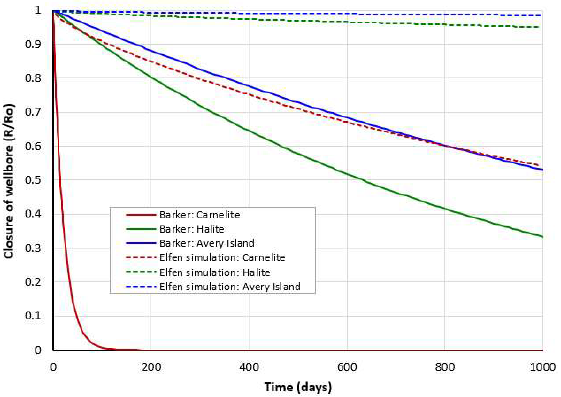Figure 3: Comparison of Elfen simulation against Barker’s solution for Carnelite, Halite and Avery Island

Closure of the wellbore as calculated using Barker’s formulation is consistently overestimated, with much higher creep rates compared to the Elfen model.  This is attributed to the diminishing effective stress in the numerical solution (Figure 4), whereas the Barker solution assumes constant effective stress.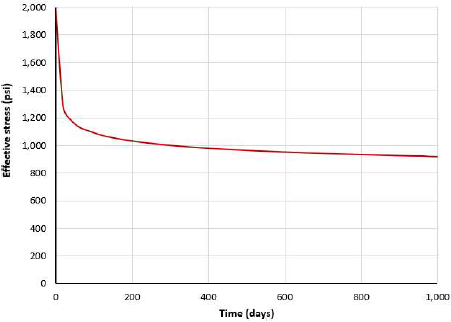Figure 4: Variation of effective stress at the wellbore for Avery Island salt

Updating the Barker’s solution with a non-constant effective stress, extracted from the Avery Island Simulation Figure 4, illustrates the conservatism of the assuming constant effective stress (Figure 5).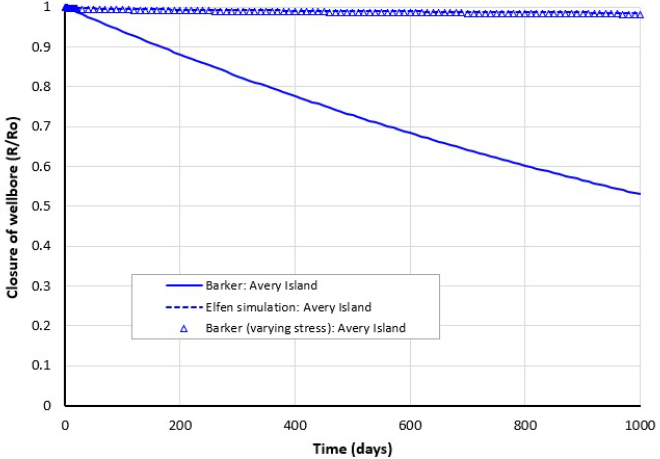Figure 5: Comparison of Elfen simulation against Barkers’ solution (constant and varying effective stress) for Avery Island

Note, the updated Barker solution is in agreement with the Elfen’s numerical solution.